# Jewelry box

The bottom of Zeyda's jewelry box is a rectangle with the length of 5 3/8 inches and a width of 3 1/4 inches. What is the area of the bottom of the jewelry box?

S =  17.4688 inch2

### Step-by-step explanation: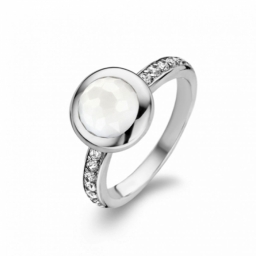Did you find an error or inaccuracy? Feel free to write us. Thank you!Tips to related online calculators
Need help to calculate sum, simplify or multiply fractions? Try our fraction calculator.

#### You need to know the following knowledge to solve this word math problem:

We encourage you to watch this tutorial video on this math problem:

## Related math problems and questions:

• A rectangle 6A rectangle measures 2 4/5 inches by 2 1/5 inches. What is its area?
• A box 2A box has a length of 4 1/2 inches, a width of 3 2/3 inches and a height of 8 1/4 inches. What is the volume of the box?
• Fractions and mixed numerals(a) Convert the following mixed numbers to improper fractions. i. 3 5/8 ii. 7 7/6 (b) Convert the following improper fraction to a mixed number. i. 13/4 ii. 78/5 (c) Simplify these fractions to their lowest terms. i. 36/42 ii. 27/45 2. evaluate the follow
• AlexandraAlexandra made a rectangular quilt the measured 3 1/4. 2 3/4 feet in width. What is the area of the quilt in square feet? Write an equation to solve.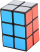A gift box has dimensions of: 8 1/2 inches, 5 1/2 inches, and 2 1/2 inches, respectively. How many cubes with side lengths of 1/2 inches would be needed to fill the gift box?
• TimothyTimothy is 56 4/5 inches tall. Theresa is 1 1/6 inches shorter than Timothy and Jane is 1 1/3 inches shorter than Theresa. How tall is Jane?
• The length 5The length and breadth of rectangular field is 3 1/5 m  and 1 3/7 m. Find the area of rectangular field.
• TorqueTorque and Mari each multiplied 1/8 inch times 5/8 inch. Tartaric 5/8 squares point inches. And Marie got 5/64 squared thought inches tall. Which student found a corrupt area?
• Thomas 2Thomas is 56 3/4 inches tall. Jillian is 1 3/8 inches shorter than Thomas and Jane is 1 1/5 inches shorter than Jillian. How tall is Jane? Label the names on the tape diagram to represent this problem. Jane Jillian Thomas Excellent!
• Length 7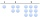Length equals 7/6 inch in width equals 7/9 inches the area is?
• A piece 3A piece of rectangle cloth has an area of 2 4/5 meters. Its breadth is 1 1/5 meters what is the length of the cloth?
• Three rectangles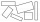Some wire is used to make 3 rectangles: A, B, and C. Rectangle B's dimensions are 3/5 cm larger than Rectangle A's dimensions, and Rectangle C's dimensions are again 3/5 cm larger than Rectangle B's dimensions. Rectangle A is 2 cm by 3 1/5 cm. What is the
• A turtle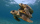A turtle is 20 5/6 inches below the surface of a pond. It dives to a depth of 32 1/4 inches. What is the change in the turtle’s position? Write your answer as a mixed number.
• Divide 11Divide the product of 4 and 5/8 by 1 1/2. Write your answer as a mixed number.
• Juila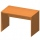Juila has a rectangular table that is 3 1/2 feet long and 1 3/4 feet wide. What is the area of Juila's table?
• A triangle 2A triangle has a base of 2 inches and a height of 2 3/4 inches. What is the area of the triangle?
• Long and wideWhat is the area of a rectangle that is 1 1/4 meters long and 3/4​ of a meter wide